# Constructing the likelihood

Before reading this section, it might be worth while – for statisticians unfamiliar with likelihood inference in the context of diffusion processes – to read the section on likelihood inference for purely diffuse processes in the DiffusionRgqd package. However, the concepts outlined here follow naturally from those outlined in the vignette on generating transition densities.

Consider a process observed discretely at time epochs $$t_1,t_2,\ldots, t_n$$ giving rise to the time series $$D_S = \{\mathbf{X}_{t_1},\mathbf{X}_{t_2},\ldots,\mathbf{X}_{t_n}\}$$. In the case of jump free diffusions we operate under the assumptions that the time series is observed without error (or at least a high degree of precision) and that the data generating process is a jump free process. Discarding the latter assumption and allowing for the data generating process to contain jumps presents subtle yet significant complications with respect to performing inference in practice. Whilst accounting for discontinuous behaviour in a diffusion model by including a jump processes in the dynamics of a model may serve to explain important features of the data at hand, it is important to note that it creates an element of inherent latency when the process of interest is observed at discrete points within a finite time horizon. This follows since we only observe the diffuse part of the underlying process in the sense that the jump part is only visible through its effect on the trajectories of the diffuse part, $$X_t$$. This latency manifests in various ways depending on the nature of the jump process and the resolution of the observed series. Collectively these difficulties amount to informational latency rather than a methodological issue. For example, in practice, it would rarely be a problem to have an immeasurably weak jump signal as the motivation for modelling real world phenomena with a jump diffusion model usually stems from a priori knowledge of the presence of ‘jump’ behaviour in an observed series (Honore 1998). This however does not preclude the quality of such a signal. Furthermore, having strong evidence for the presence of jump does not circumvent the need for a sufficiently long time series. This stems from the fact that we have to distil both the rate at which jumps occur (long run dynamics) as well as the distributional qualities of the jumps (instantaneous dynamics) from the distorted signal - whatever the data resolution may be.

Given observations $$D_S$$ and some jump diffusion model parametrized by the vector $$\boldsymbol\theta$$, we can formulate the likelihood mathematically from the transitional density using the usual Markov arguments: $L(\boldsymbol\theta|D_S) \propto \prod_{i=1}^{n-1} f(\textbf{X}_{t_{i+1}}|\textbf{X}_{t_{i}},\boldsymbol\theta).$ Subsequently applying the excess factorization (see the vignette on generating transition densities for more details on the factorization), we can write the likelihood as: \begin{aligned} L(\boldsymbol\theta|D_S) \propto& \prod_{i=1}^{n-1}\bigg( P(\textbf{N}_{t_{i+1}}-\textbf{N}_{t_{i}}=0)f_D(\textbf{X}_{t_{i+1}}|\textbf{X}_{t_{i}},\boldsymbol\theta)+P(\textbf{N}_{t_{i+1}}-\textbf{N}_{t_{i}}>0)f_E(\textbf{X}_{t_{i+1}}|\textbf{X}_{t_{i}},\boldsymbol\theta)\bigg).\\ \end{aligned} Using this formulation, the ability to perform inference on jump diffusions hinges on the data being of sufficiently high resolution and/or the jump signal being strong enough at the given resolution, in order for the information about the jump process to manifest as the contrast between the diffuse and the excess distributions $$f_D(.)$$ and $$f_E(.)$$ respectively. Fortunately, as is the nature of datasets to which jump models are applied, that presence of a jump signal is usually what motivates the use of a jump diffusion model in the first place. Thus, using the moment truncation methodology in conjunction with the excess factorization, it is possible to perform inference on a wide range of non-linear, time-inhomogneous jump diffusion models.

# Simulated diffusion processes

## CIR process with state-dependent intensity and Normal jump distribution

Consider a jump diffusion governed by the parametrised SDE:

\begin{aligned} dX_t &= \theta_1(\theta_2-X_t)dt +\theta_3\sqrt{X_t}dB_t +dP_t\\ dP_t &= \dot{z}_t dN_t \end{aligned}

with intensity $$\lambda(X_t,t) = \theta_4 X_t$$ and where $$\dot{z}_t \sim \mbox{N}(\theta_5,\theta_6^2)$$. For conveinince we have included a simulates trajectory of this process under the parameter set $$\boldsymbol\theta = \{1,5,0.1,0.25,0.5,0.5\}$$. Subsequently, we can run the experiment using the R code:

library(DiffusionRjgqd)
data(JSDEsim1)
attach(JSDEsim1)
plot(JSDEsim1$Xt~JSDEsim1$time,type='l',col='blue',xlab='Time (t)',ylab=expression(X[t]),main='Simulated trajectory')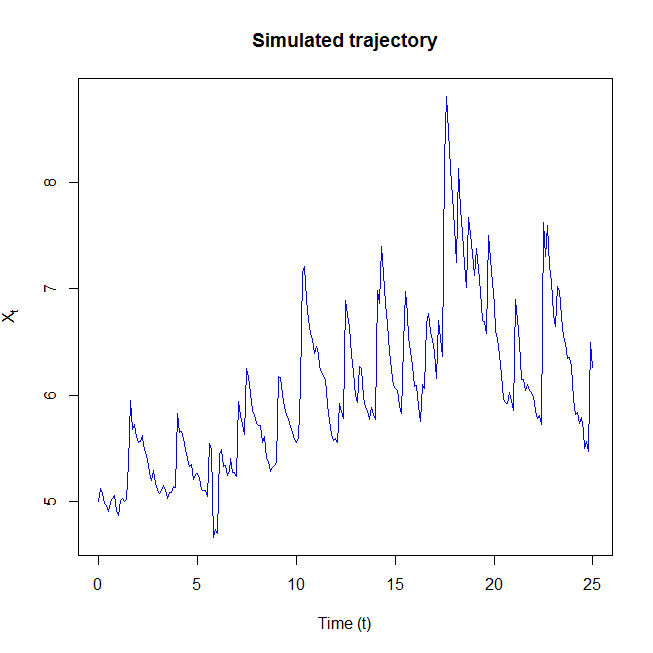Here, JSDEsim1 denotes a simulated dataset where a single trajectory of the model process is simulated and subsequently recorded at equispaced points along a finite observation horizon. For this dataset, $$X_t$$ is observed at times $$t_1 =0, t_2 = 0.1,\ldots,t_{251}=25$$. In order to estimate parameters for the simulated jump diffusion we can define the model using the JGQD-syntax and call the JGQD.mcmc() which maximizes the likelihood via the random walk Metropolis-Hastings algorithm (RWMH). This should be familiar from the DiffusionRgqd. For purposes of the analysis we place priors on the parameters $$\theta_4$$ and $$\theta_6$$ where: \begin{aligned} \theta_4 &\sim \mbox{Gamma}(0.001,0.001),\\ \theta_6^2 &\sim \mbox{InvGamma}(0.001,0.001).\\ \end{aligned}

In R:

# Define the model:
JGQD.remove()
G0 <- function(t){theta*theta}
G1 <- function(t){-theta}
Q1 <- function(t){theta*theta}
Jmu  <- function(t){theta}
Jsig <- function(t){theta}
Lam1 <- function(t){theta}
priors <- function(theta)
{
dgamma(theta,0.001,0.001)*dgamma(1/theta^2,0.001,0.001)
}

# Define some starting parameters and run the MCMC:
burns   <- 10000
theta   <- c(10,10,2,2,0,1)
sds     <- c(0.154,0.171,0.037,0.156,0.155,0.2)/4
model_1 <- JGQD.mcmc(JSDEsim1$Xt,JSDEsim1$time,mesh=20,theta=theta,sds=sds,
updates=updates,burns=burns)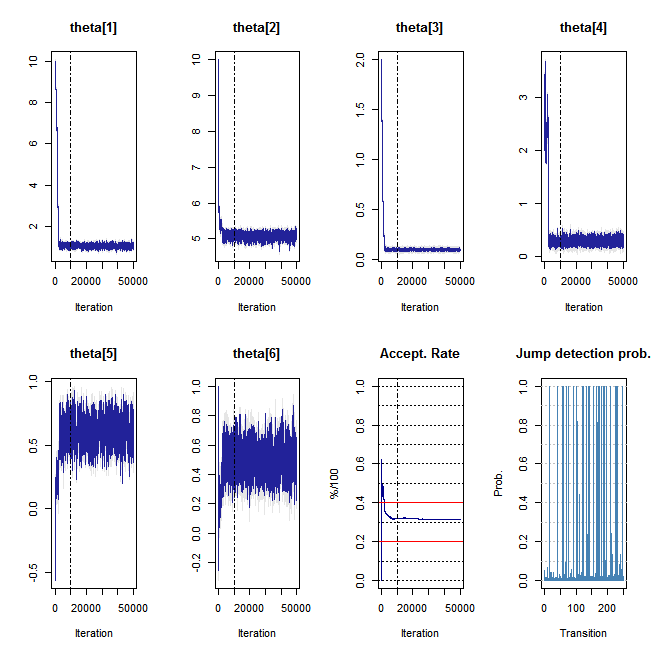================================================================
================================================================
_____________________ Drift Coefficients _______________________
G0 : theta*theta
G1 : -theta
G2
___________________ Diffusion Coefficients _____________________
Q0
Q1 : theta*theta
Q2
_______________________ Jump Components ________________________
Lam0
Lam1 : theta
........................... Jumps ..............................
Normal
Jmu : theta
Jsig : theta
__________________ Distribution Approximant ____________________
Trunc. Order    : 4
Dens.  Order    : 4
=================================================================

_______________________ Jump Components ________________________
Time Homogeneous    : Yes
Data Resolution     : Homogeneous: dt=0.1
# Removed Transits. : None
Density approx.     : 4 Ord. Truncation +4th Ord. Saddlepoint Appr.
Elapsed time        : 00:04:12
...   ...   ...   ...   ...   ...   ...   ...   ...   ...   ...
dim(theta)          : 6
DIC                 : -267.962
pd (eff. dim(theta)): 5.708
---------------------------------------------------------------- 

In order to calculate parameter estimates from the resulting MCMC output, we make use of the JGQD.estimates() function:

JGQD.estimates(model_1,thin=200,burns)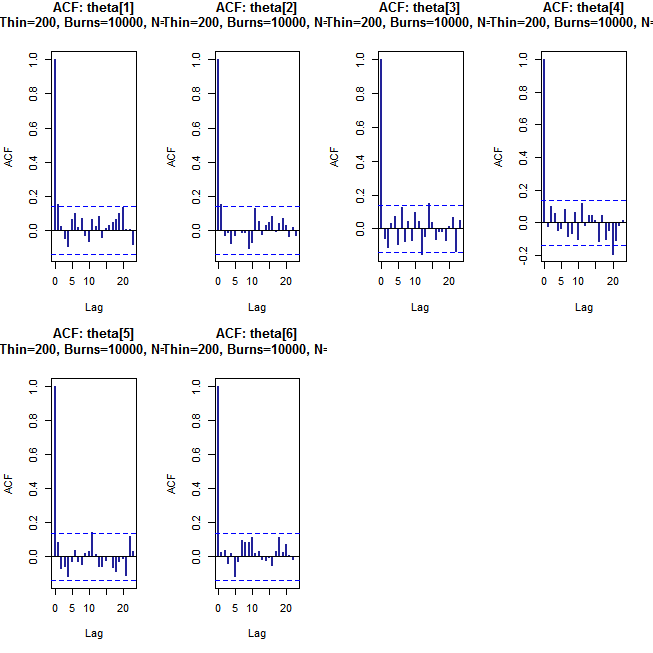Estimate Lower_CI Upper_CI
theta    1.046    0.899    1.200
theta    5.072    4.928    5.190
theta    0.100    0.091    0.109
theta    0.271    0.193    0.367
theta    0.616    0.449    0.785
theta    0.502    0.358    0.635

One of the features of the methodology which underpins the package is that it affords the opportunity to handle the unobserved jump sequence (to an extent). During execution of the MCMC algorithm, JGQD.mcmc() will attempt to decode and estimate the probability of each transition containing a jump. This can be acccessed via the model_1$decode.prob vactor. For comparison we have included the true sequnce of transitions which contain jumps. Subsequently we compare the estimated and true jump sequences: plot(JSDEsim1$contains_jump[-1],type='n',ylim=c(0,1.2),axes=F,
main='True vs. Est. Jump Sequence',xlab='Transition',ylab='')
abline(v=which(JSDEsim1$contains_jump[-1]==1),col='gray75',lty='dashed') lines(model_1$decode.prob,type='h',col='blue')
axis(1)
axis(2,seq(0,1,1/2))
legend('topright',legend = c('True jump transitions','Est. prob. of jump'),col=c('gray75',4),bty='o',lty=c('dashed','solid'),bg='white')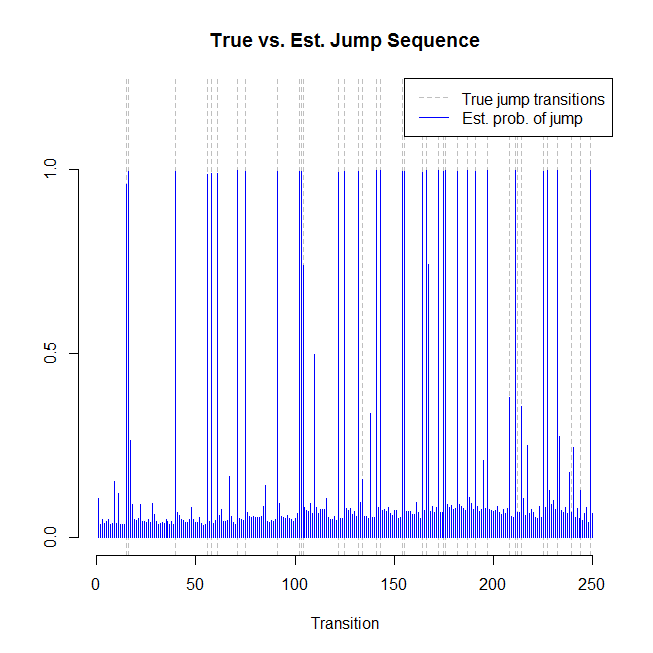For the simulated dataset, we can to a reasonable degree of accuracy decode which observed transitions most likely contain jumps. Naturally, the sequence of probabilities are based on the model being used, so results may vary, but asssuming that the jump signal is sufficiently strong we should be able to detect a good number of jumps in the data.

## A bivariate non-linear, coupled process with bivariate Normal jump distribution

Following along the lines of the previous example, we investigate a simulated a bivariate jump diffusion process. Using similar techniques to the scalar case we can accurately approximate the transitional density of a biavariate jump diffusion. Using the transition density approximation in conjunction with the likelihood expression we can perform inference just as in the scalar case. For purposes of the demonstration we consider a non-linear time homogeneous model of the form:

\begin{aligned} dX_t &= 0.5(2+Y_t-X_t)dt+0.1\sqrt{X_tY_t}dB_t^1+dP_t^1\\ dY_t &= (5-X_t)dt+0.1\sqrt{Y_t}dB_t^2+dP_t^2\\ \end{aligned} where

\begin{aligned} dP_t^1&=\dot{z}_1dN_t^1\\ dP_t^2&=\dot{z}_2dN_t^1\\ \end{aligned}

with intensity $$\lambda(X_t,Y_t) = 1$$ and where $\{\dot{z}_1,\dot{z}_2\}^\prime\sim\mbox{Bivariate Normal}(\{0.5,0.5\}^\prime,\mbox{diag}(\{0.5^2,0.5^2\}^\prime)).$ For convenience we have included a simulated dataset for this model which can be called using data(JSDEsim2). Additionally, the corresponding sequence of jumps and jump arrival times were recorded in the dataset JSDEsim3. Although the true jump sequence plays no role in performing inference using the approximate likelihood function, it is useful to compare the parameter estimates that result from the indirect estimates of the jump mechanism parameters (indirectly observed via the jump diffusion) to estimates based on the true jump realisations. Subsequently, we can run the experiment using the R code:

library(DiffusionRjgqd)
data(JSDEsim2)
attach(JSDEsim2)
data(JSDEsim3)
attach(JSDEsim3)

plot(JSDEsim2$Xt~JSDEsim2$time,type='l',col='#BBCCEE',ylim=c(-3,13),xlim=c(0,60),axes=F,main='Simulated Trajectory',xlab = 'Time',ylab ='X_t')
lines(JSDEsim2$Yt~JSDEsim2$time,type='l',col='#222299')

axis(1,at=seq(0,50,5))
axis(1,at=seq(0,50,5/5),tcl=-0.2,labels=NA)
axis(2,at=seq(-3,13,1))
axis(2,at=seq(-3,13,1/5),tcl=-0.2,labels=NA)

lines(Xjumps~Jtime,type='h',col='#BBCCEE')
lines(Yjumps~Jtime,type='h',col='#222299')
mx=mean(Xjumps)
sx=sd(Xjumps)
my=mean(Yjumps)
sy=sd(Yjumps)
segments(50,-5,50,9,lty='dotted')
xx=seq(-3,3,1/10)
yy=dnorm(xx,mx,sx)
yy = (yy-min(yy))/(max(yy)-min(yy))*9+51*1
lines(xx~yy,col='#BBCCEE')
yy=dnorm(xx,my,sy)
yy = (yy-min(yy))/(max(yy)-min(yy))*9+51*1
lines(xx~yy,col='#222299')

text(55,10.0,substitute(hat(theta)==a,list(a=round(mx,2))),cex=0.8)
text(55,9.0,substitute(hat(theta)==a,list(a=round(sx,2))),cex=0.8)
text(55,8.0,substitute(hat(theta)==a,list(a=round(my,2))),cex=0.8)
text(55,7.0,substitute(hat(theta)==a,list(a=round(sy,2))),cex=0.8)
abline(h=0,lty='dotted')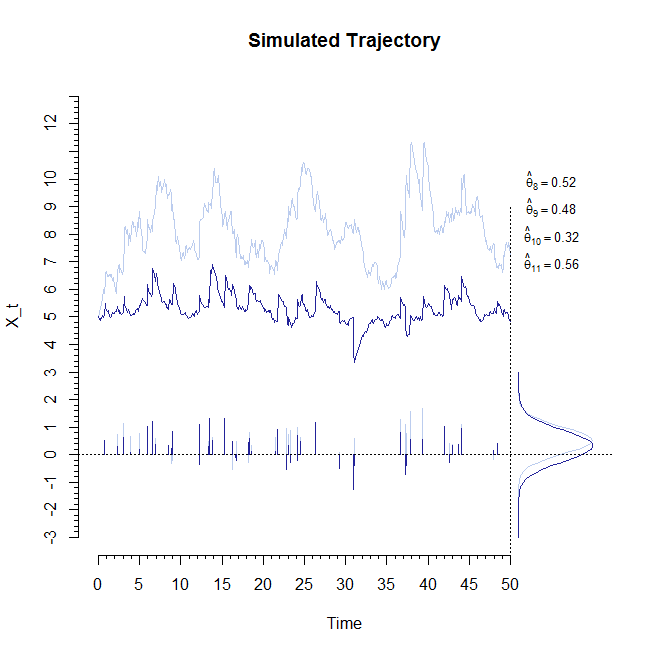The figure above shows a simulated trajectory for the jump diffusion ($$X_t$$ in lightblue and $$Y_t$$ in dark blue) and the corresponding sequence of jumps. From the jump sequence we calculate parameter estimates for the jump distribution parameters and superimpose the resulting density functions in the right peripheral of the plot. Note that, despite the true parameters for the marginals of the jump distribution being equal, the estimates calculated from the directly observed jump sequence differ slightly. Specificlally, the parameter estimate for theta is somewhat lower than the true value. This seems to suggest that the sequence of jumps may contain values which are improbable (but not impossible) under the true model. Now, for purposes of the experiment we can perform inference via the jump diffusion (in which case, again, the true jump sequence is not used in the likelihood evaluation directly but only through its effect on the trajectory of the jump diffusion) and compare the resulting estimates to that of the true parameter set:

X=cbind(JSDEsim2$Xt,JSDEsim2$Yt)

# Define the model:
JGQD.remove()
a00 <-function(t){theta*theta}
a10 <-function(t){-theta}
a01 <-function(t){theta}
c11 <-function(t){theta*theta}

b00 <-function(t){theta*theta}
b01 <-function(t){-theta}
f01 <-function(t){theta*theta}

# Constant intensity
Lam00= function(t){theta}

# Normal jumps:
Jmu1   <-function(t){theta}
Jmu2   <-function(t){theta}
Jsig11 <-function(t){theta*theta}
Jsig22 <-function(t){theta*theta}

# Some starting parameters:
theta  <-c(rep(1,11))
sds    <-c(0.08,0.22,0.01,0.04,0.16,0.01,0.10,0.07,0.09,0.05,0.09)/2
burns  <-10000

res <-BiJGQD.mcmc(X,JSDEsim2time,mesh=10,theta,sds,updates,burns=burns)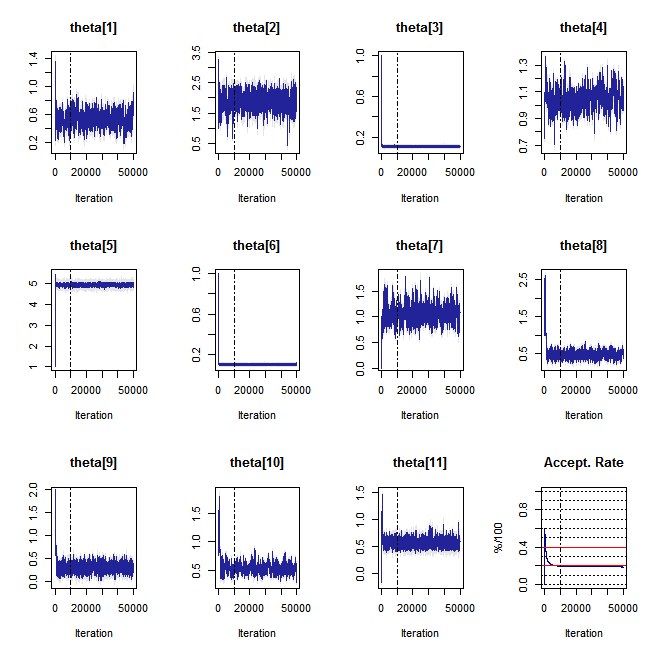Compiling C++ code. Please wait. ================================================================ GENERALIZED QUADRATIC DIFFUSON ================================================================ _____________________ Drift Coefficients _______________________ a00 : theta*theta a10 : -theta a01 : theta ... ... ... ... ... ... ... ... ... ... ... b00 : theta*theta b01 : -theta ___________________ Diffusion Coefficients _____________________ c11 : theta*theta ... ... ... ... ... ... ... ... ... ... ... ... ... ... ... ... ... ... ... ... ... ... ... ... ... ... ... ... ... ... ... ... ... f01 : theta*theta _______________________ Jump Components ________________________ ......................... Intensity ............................ Lam00 : theta ........................... Jumps .............................. Jmu1 : theta Jmu2 : theta Jsig11 : theta*theta Jsig22 : theta*theta _____________________ Prior Distributions ______________________ d(theta):None. ================================================================ _______________________ Model/Chain Info _______________________ Chain Updates : 50000 Burned Updates : 10000 Time Homogeneous : Yes Data Resolution : Homogeneous: dt=0.1 # Removed Transits. : None Density approx. : 4th Ord. Truncation, Bivariate-Saddlepoint Elapsed time : 00:14:28 ... ... ... ... ... ... ... ... ... ... ... dim(theta) : 11 DIC : -855.021 pd (eff. dim(theta)): 10.704 ---------------------------------------------------------------- JGQD.estimates(res,thin=200,burns=burns)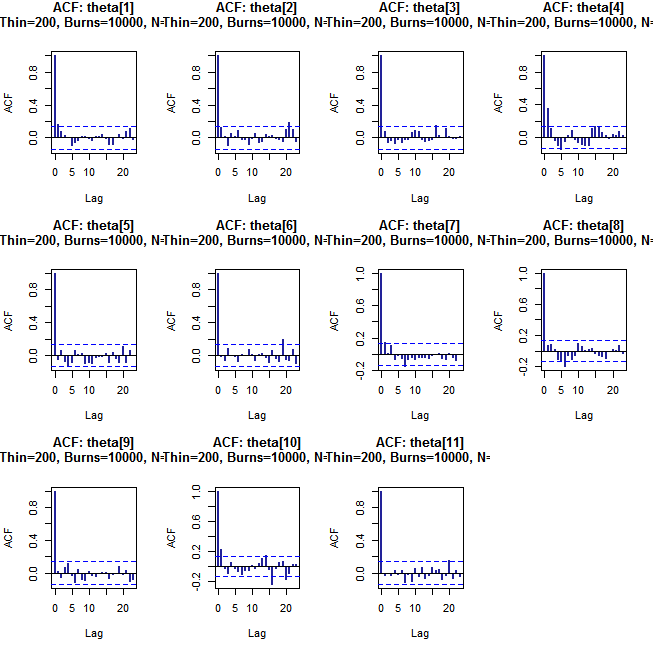Estimate Lower_CI Upper_CI theta 0.535 0.357 0.694 theta 1.889 1.458 2.253 theta 0.109 0.102 0.114 theta 1.046 0.923 1.192 theta 4.958 4.878 5.035 theta 0.106 0.099 0.112 theta 1.061 0.809 1.364 theta 0.479 0.315 0.625 theta 0.314 0.171 0.459 theta 0.528 0.405 0.673 theta 0.560 0.463 0.674 Note that the parameter estimates are quite accurate and that the lower estimate for theta is preserved in the jump diffusion parameter estimates. This serves to highlight that even under ideal conditions (the dataset was reasonably long and observed at reasonably high resolution) it is possible for the observed series to contain unllikely observations under the true data generating process. Consequently, it is important when interpreting parameter estimates to understand how the data resolution effects the analysis and how some elements of the model may be difficult to pin down. # A jump diffusion model of Google equity volatility Perhaps the most cited use of jump diffusion models are in the field of mathematical finance. An important application of jump diffusion processes in finance is the modeling of volatility processes. One of the most famous of these is the S&P 500 volatility index (VIX), which serves as a measure of volatility for an index of the S&P 500 index of securities. Although the S&P 500 has been the subject of numerous empirical studies, highly traded individual securities have enjoyed similar interest in recent times. Indeed, the Chicago Board Options Exchange (CBOE) now provides equity volatility indices for a couple of hoghly traded stocks that are based on similar methods as the VIX. For purposes of this example we consider the equity volatility of internet search giant, Google. Using the Quandl package (McTaggart and Daroczi 2015), we can source data in R for Google equity volatility (VXGOG) directly:  library(DiffusionRjgqd) library(Quandl) # Source data for the Google equity volatility: quandldata1 <- Quandl("CBOE/VXGOG", collapse="daily", start_date="2010-03-11",end_date="2016-01-01", type="raw") Vt <- rev(quandldata1[,names(quandldata1)=='Close']) time1 <-rev(quandldata1[,names(quandldata1)=='Date']) # Make a plot of the data plot(Vt~time1,type='l',col='#222299',ylim=c(10,55),main='Google Equity Volatility (VXGOG)', xlab = 'Time',ylab ='Volatility %',lwd=1)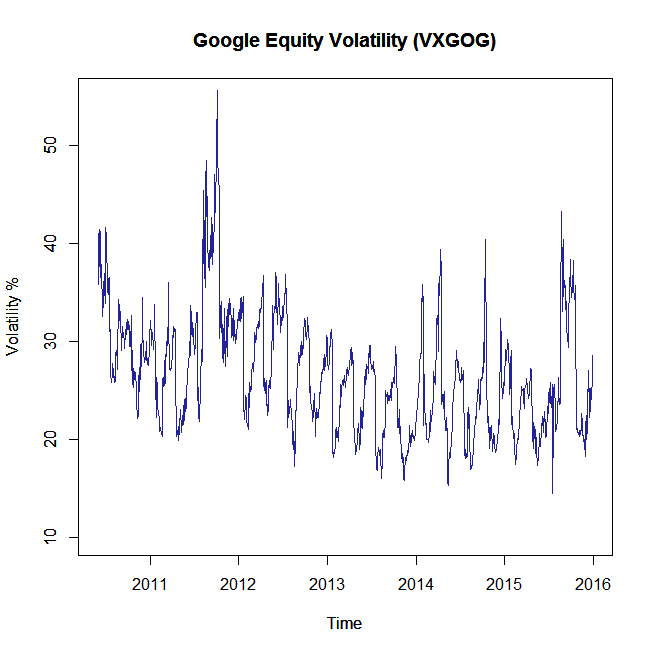We source daily observations for the time period starting 2013 to end of 2015. In order to model the series we define a jump diffusion model of the form: \begin{aligned} dX_t &= \theta_1\big(\theta_2+\theta_7\sin\big(8\pi t + (\theta_8-0.5) 2 \pi\big)\big)-X_t)dt+\theta_3X_tdB_t +dP_t\\ dP_t &= \dot{z}_tdN_t\\ \end{aligned} where $$\lambda(X_t,t) = \theta_4X_t$$ and $$\dot{z}_t\sim \mbox{N}(\theta_5,\theta_6^2)$$. Note that this is a relatively complicated model. Typically such an analysis would consist of fitting a plethora of models and finding a most likeliy candidate based on model fit statistics. However, this can be a lengthy process and we cut to the chase for this example (a more in-depth study of this dataset can be found in the methodology paper). The model presented here contains a cyclical drift component corresponding to a quarterly volatility cycle, state-dependent volatility (i.e., the volatility of volatility varies in accordance with the level of the process) and state-dependent jump intensity. That is, we postulate that the arrival rate for jump events varies in accordance with the level of volatility. Consequently jumps are more likely when volatility is high as opposed to when it is low. In order to facilitate the estimation procedure we place some prior distributions on the parameter space: \begin{aligned} \theta_1 &\sim \mbox{Gamma}(0.001,0.001)\\ \theta_2 &\sim \mbox{N}(25,5^2)\\ \theta_3^2 &\sim \mbox{InvGamma}(0.001,0.001)\\ \theta_4 &\sim \mbox{Gamma}(0.001,0.001)\\ \theta_6^2 &\sim \mbox{InvGamma}(0.001,0.001)\\ \theta_7 &\sim \mbox{Gamma}(0.001,0.001)\\ \theta_8 &\sim \mbox{Beta}(0.5,0.5).\\ \end{aligned} Note that the priors are mostly uninformative, however, they do serve to restrict relevant parameters to appropriate domains. For example, $$\theta_8$$ is restricted to the interval $$[0,1]$$ since the likelihood would contain multiple identical modes otherwise. Using the JGQD.mcmc() we can fit the jump diffusion process to the observed series and calculate parameter estimates:  JGQD.remove() G0 <- function(t){theta*theta + theta*theta*sin(8*pi*t + (theta - 0.5)*2*pi)} G1 <- function(t){-theta} Q2 <- function(t){theta*theta} Lam1<- function(t){theta} Jmu <- function(t){theta} Jsig<- function(t){theta} priors<-function(theta) { rs =dgamma(theta, 0.001, 0.001)* dnorm(theta, 25, 5)* dgamma(1/theta^2, 0.001, 0.001)* dgamma(theta, 0.001, 0.001)* dgamma(1/theta^2, 0.001, 0.001)* dgamma(theta, 0.001, 0.001)* dbeta(theta,0.5,0.5) if(is.na(rs)){return(0.000000000001)} return(rs) } X <- Vt time <- cumsum(c(0, diff(as.Date(time1))*(1/365))) updates <- 100000 burns <- 25000 theta <- c(50, 50, sqrt(5), 5, 5, 5, 50, 0.1) sds <- c(1.87, 2.96, 0.02, 0.17, 0.39, 0.40, 0.71, 0.03)/1.5 model_1 <- JGQD.mcmc(X, time, 5, theta, sds, updates, burns)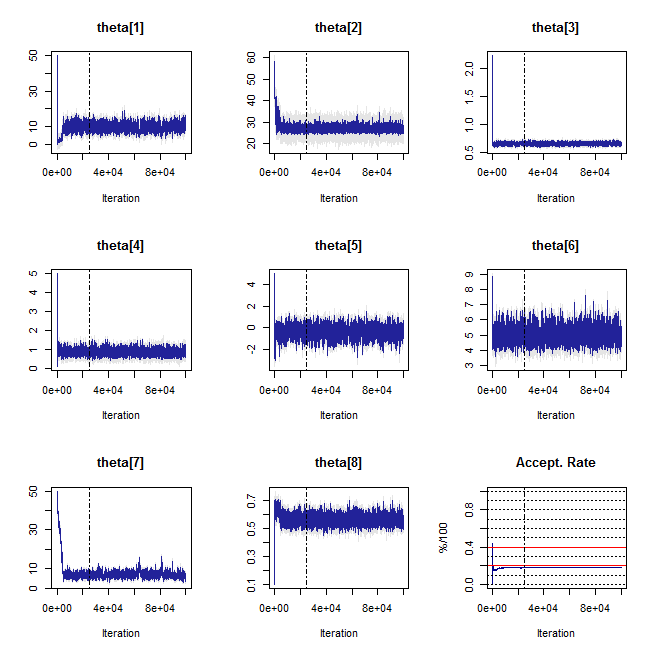================================================================ Jump Generalized Quadratic Diffusion (JGQD) ================================================================ _____________________ Drift Coefficients _______________________ G0 : theta*theta+theta*theta*sin(8*pi*t+(theta-0.5)*2*pi) G1 : -theta G2 ___________________ Diffusion Coefficients _____________________ Q0 Q1 Q2 : theta*theta _______________________ Jump Components ________________________ Lam0 Lam1 : theta ........................... Jumps .............................. Normal Jmu : theta Jsig : theta __________________ Distribution Approximant ____________________ Density approx. : Saddlepoint Trunc. Order : 4 Dens. Order : 4 ================================================================= _______________________ Jump Components ________________________ Chain Updates : 1e+05 Burned Updates : 25000 Time Homogeneous : No Data Resolution : Variable: min(dt)=0.0027, max(dt)=0.0137 # Removed Transits. : None Density approx. : 4 Ord. Truncation +4th Ord. Saddlepoint Appr. Elapsed time : 00:23:49 ... ... ... ... ... ... ... ... ... ... ... dim(theta) : 8 DIC : 4751.671 pd (eff. dim(theta)): 7.99 ----------------------------------------------------------------  # Calculate parameter estimates: ests <- JGQD.estimates(model_1, thin = 200, burns) ests   Estimate Lower_CI Upper_CI theta 9.811 6.547 13.069 theta 27.166 25.747 28.828 theta 0.661 0.629 0.691 theta 0.844 0.627 1.105 theta -0.370 -1.225 0.502 theta 5.038 4.313 5.905 theta 7.077 5.050 9.846 theta 0.566 0.518 0.617  From a modeling perspective, the use of a jump diffusion can be made clear by comparing the model fit to traditional diffusion models. For example, compared to its jump-free counterpart – a time-inhomogeneous CIR model of the equity volatility (here, the DiffusionRgqd . package can be used to fit the jump-free model):  # Purely diffuse process: library(DiffusionRgqd) GQD.remove() G0 <- function(t){theta*theta + theta*theta*sin(8*pi*t + (theta - 0.5)*2*pi)} G1 <- function(t){-theta} Q2 <- function(t){theta*theta} priors <- function(theta) { rs =dgamma(theta, 0.001, 0.001)* dnorm(theta, 25, 5)* dgamma(1/theta^2, 0.001, 0.001)* dgamma(theta, 0.001, 0.001)* dbeta(theta, 0.5, 0.5) if(is.na(rs)){return(0.000000000001)} return(rs) } theta <- c(50, 50, sqrt(5), 10, 0.1) sds <- c(2.87, 2.96, 0.02, 1.21, 0.03)/2 model_2 <- GQD.mcmc(X, time, 5, theta, sds, updates, burns, print.output = FALSE) a comparison of DIC reveals a substantial improvement in fit:  # Compare DIC values: JGQD.dic(list(model_1, model_2))  Elapsed_Time Time_Homogeneous p DIC pD N Model 1 00:23:49 No 8.00 [=] 4751.67 7.99 1409 Model 2 00:08:24 No 5.00 5449.00 5.17 1409 Although the parameter estimates can give an indication as to the dynamics of the jump mechanism of the process, the JGQD.mcmc() function provides a usefull statistic for assessing the probability of jump events. From the model output we can access the list variable zero.jump which gives the estimated mean probability of observing at least one jump at each iteration of the MCMC run. We may thus draw a frequency histogram of said probability in order to gain insiight into the fitted likelihood of a jump arrival for a typical transition.

 # Make a histogram of the mean jump probability per transition horizon:
hist(model_1$zero.jump[seq(burns,updates,1)], freq= FALSE, col='gray74', border = 'white', xlab = 'Probability', main = 'Mean Jump Probability per Transition')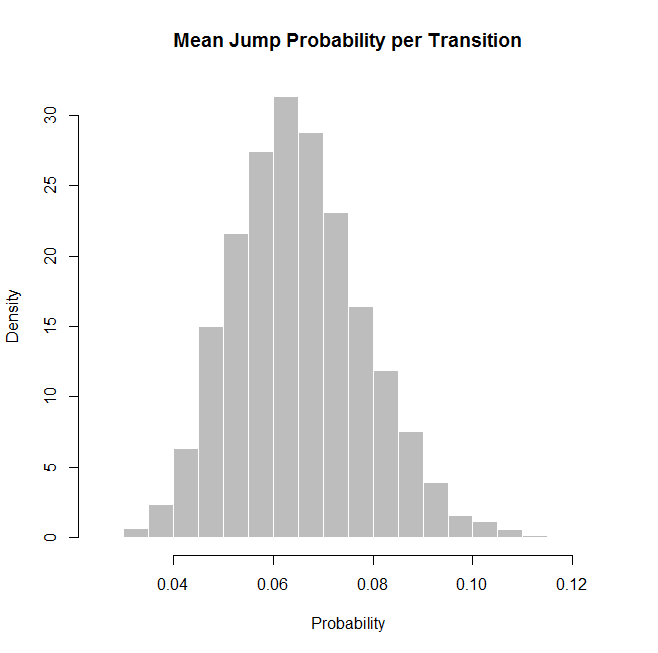Based on the histogram we can expect to see at least one jump in volatility on any given trading day with a probability of around 6.5%. For reference we have also superimposed a decoded sequence of jump probabilities for each transition horizon. That is, we estimate the probability of each observed transition containing a jump (based on the underlying model) and artificially select pre(post?)dicted jump arrivals for the onserved time series. This is done by imposing the euristic rule that a a 90% or higher estimated probability (contained in the model output list variable $decode.prob) of containing a jump is deemed to be difinitive in detecting a jump event. Although this is not by any means rigourous it is useful for exploratory analysis. Visually, the estimated arival times appear to coincide with large movements in the volatility series. Specifically, some of the decoded arrivals appear to be structural in nature where drops in volatility are observed after cyclical increases in volatility… More in-depth analysis of this to follow in upcoming research ,but consider the following:

plot(model_1$decode.prob~time1[-1], type='h', ylim = c(0, 1), xlab ='Time (t)', ylab= 'Prob.',main ='Detection probability sequence: VXGOG',col = 'steelblue') abline(h=0.8,lty='dotted')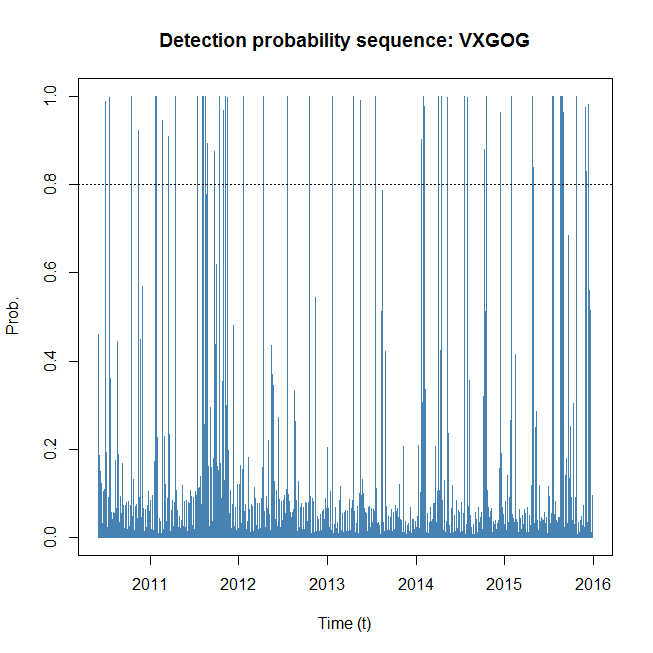# Earnings report dates (appr): QERs<-c("2010-07-16", "2010-10-14", "2011-01-20", "2011-04-14", "2011-07-14", "2011-10-13", "2012-01-19", "2012-04-12", "2012-07-19", "2012-10-18", "2013-01-22", "2013-04-18", "2013-07-18", "2013-10-17", "2014-01-30", "2014-04-16", "2014-07-17", "2014-10-16", "2015-01-29", "2015-04-23", "2015-07-16", "2015-10-22") QS <- c(2, 3, 4, 1, 2, 3, 4, 1, 2, 3, 4, 1, 2, 3, 4, 1, 2, 3, 4, 1, 2, 3) plot(Vt~time1, type = 'l', col = '#222299', ylim=c(10, 55), main='Google Equity Volatility (VXGOG)', xlab = 'Time', ylab = 'Volatility %') at.dates <- c(time1[-1])[which(model_1$decode.prob > 0.8)]

for(i in 1:length(at.dates))
{
segments(at.dates[i], 10, at.dates[i], Vt[which(time1==at.dates[i])], col='grey30',lty='dashed')
}
QERdates <- as.Date(QERs)
for(i in 1:length(at.dates))
{
segments(QERdates[i], 10, QERdates[i], 15, col = 'red', lty = 'solid', lwd = 2)
}
text(QERdates, 9.5, labels = paste0('Q', QS), cex = 0.6)
legend('topright', legend = c('Detections (80% rule)', 'QER dates'), lty=c('dashed', 'solid'),
col = c('grey30', 'red'), lwd=c(1, 2), bg = 'white')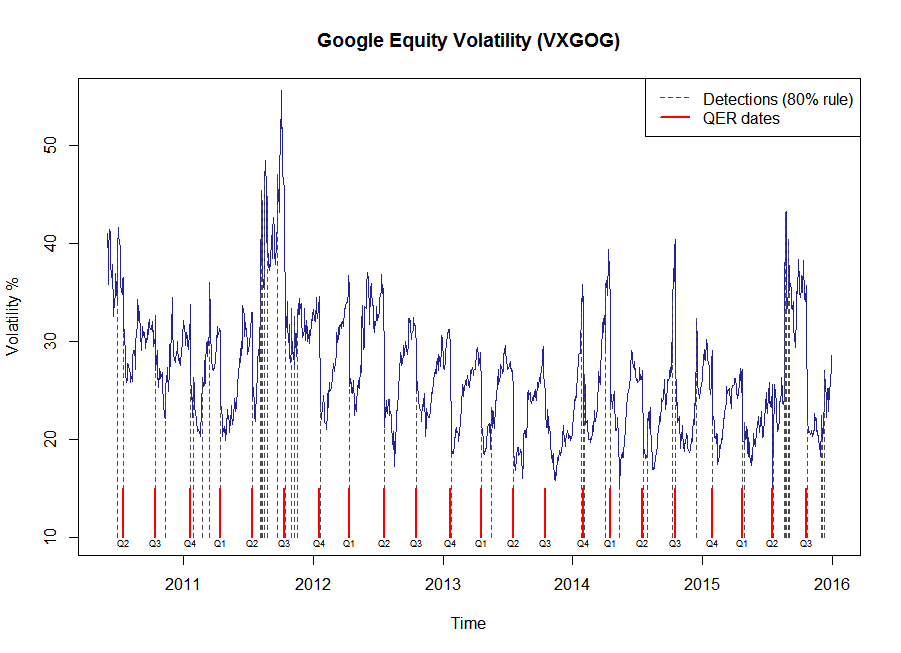browseVignettes('DiffusionRjgqd')